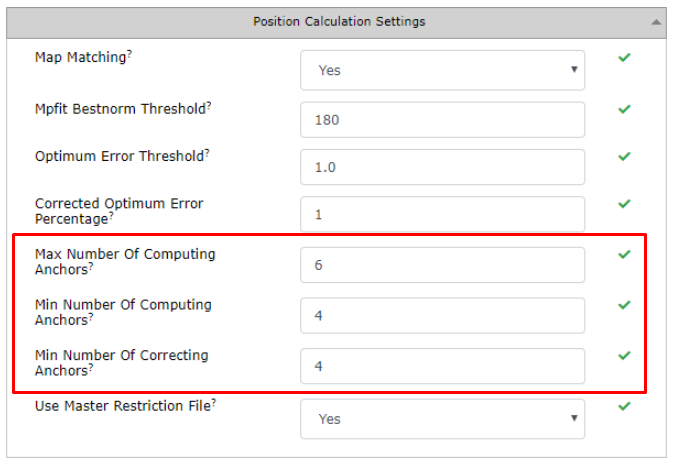# Position Calculation Settings

The RTLS Studio has got a default setting regarding minimum and maximum number of anchors used for position calculation. In some cases to optimize the RTLS System performance, could be number of Computing and correction anchors changed.## Max Number of Computing Anchors

Defines a maximum number of anchors used for position calculation.

In practice, In practice, in indoor positioning tag usually has unobstructed vision to 4-6 anchors, others are non-line-of-sight that might worsen the results. Therefore, the default value is 6. Therefore, the default value is 6. If there is good signal from more than 6 anchors, RTLS Server tries to select the best six (in terms of signal, synchronization, geometry, etc.) to calculate position.

If there is set up more then 6 anchors for computing, it consumes more CPU and time without higher benefit.

## Min Number of Computing Anchors

Defines a minimum number of anchors used for position calculation.

Mathematically, minimum is 3, however 4th anchor adds redundancy and ability to calculate the error of computation. The default is 4.

## Min Number of Correcting Anchors

Defines a minimum number of anchors used for recalculation of the position. The default is 4.